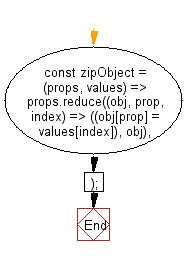# JavaScript: Return the object associating the properties to the values of a given array of valid property identifiers and an array of values

## JavaScript fundamental (ES6 Syntax): Exercise-101 with Solution

Write a JavaScript program to return the object associating the properties to the values of a given array of valid property identifiers and an array of values.

Note: Since an object can have undefined values but not undefined property pointers, the array of properties is used to decide the structure of the resulting object using Array.reduce().

Associates properties to values, given array of valid property identifiers and an array of values.

• Use Array.prototype.reduce() to build an object from the two arrays.
• If the length of props is longer than values, remaining keys will be undefined.
• If the length of values is longer than props, remaining values will be ignored.

Sample Solution:

JavaScript Code:

``````//#Source https://bit.ly/2neWfJ2
const zipObject = (props, values) =>
props.reduce((obj, prop, index) => ((obj[prop] = values[index]), obj), {});
console.log(zipObject(['a', 'b', 'c'], [1, 2]));
console.log(zipObject(['a', 'b'], [1, 2, 3]));
```
```

Sample Output:

```{"a":1,"b":2}
{"a":1,"b":2}
```

Pictorial Presentation:Flowchart:Live Demo:

See the Pen javascript-basic-exercise-101-1 by w3resource (@w3resource) on CodePen.

Improve this sample solution and post your code through Disqus

What is the difficulty level of this exercise?

Test your Programming skills with w3resource's quiz.

﻿

## JavaScript: Tips of the Day

Chunks an array into n smaller arrays

Example:

```const tips_chunkIntoN = (arr, n) => {
const size = Math.ceil(arr.length / n);
return Array.from({ length: n }, (v, i) =>
arr.slice(i * size, i * size + size)
);
}
console.log(tips_chunkIntoN([1, 2, 3, 4, 5, 6, 7,8], 4));
```

Output:

```[[1,2],[3,4],[5,6],[7,8]]
```